# Quoting in programming languages

I met the task by solving exercises from the book Structure and Interpretation of Computer Programs). It is usually called SICP (read sik-pi) is an abbreviation of the name in English.

Section 2.3 is dedicated to citation in LISP and symbolic calculations.

Ordinary – non-symbolic – calculations are reduced to calculations using arithmetic operations. For example, if I ask you to calculate the derivative of a function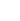at the point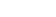you can do it according to the formula for some not very large value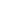.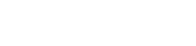Customizingwe can calculate the derivative with good accuracy.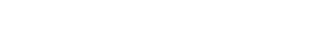Symbolic calculations, on the other hand, allow us to apply the rules for deriving derivatives and get the value absolutely exactly.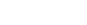Atthe value of the derivative will be exactly equal to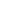.

## Implementation in Scheme

SICP proposes to calculate the derivative using citations. In English, this mechanism is called quotation.

If we enter any expression into the Scheme interpreter, it evaluates it immediately.

(+ (/ 1 1) (/ 1 1) (/ 1 2) (/ 1 6) (/ 1 24) (/ 1 120) (/ 1 720) (/ 1 5040))
; => 2.7182539682539684

But if we anticipate it quotation mark (quote), Scheme stores the expression as a list without evaluating it.

'(+ (/ 1 1) (/ 1 1) (/ 1 2) (/ 1 6) (/ 1 24) (/ 1 120) (/ 1 720) (/ 1 5040))
; => (+ (/ 1 1) (/ 1 1) (/ 1 2) (/ 1 6) (/ 1 24) (/ 1 120) (/ 1 720) (/ 1 5040))

Thus, we get a correct expression in LISP and can process it like any other list, in particular, transform it according to the rules for calculating the derivative.

Here is a simple function that plots the derivative of sums and products.

(define (variable? x) (symbol? x))
(define (same-variable? v1 v2)
(and (variable? v1) (variable? v2) (eq? v1 v2)))
(define (make-sum a1 a2) (list '+ a1 a2))
(define (make-product m1 m2) (list '* m1 m2))
(define (sum? x)
(and (pair? x) (eq? (car x) '+)))
(define (product? x)
(and (pair? x) (eq? (car x) '*)))

(define (deriv exp var)
(cond ((number? exp) 0)
((variable? exp)
(if (same-variable? exp var) 1 0))
((sum? exp)
(deriv (augend exp) var)))
((product? exp)
(make-sum
(make-product (multiplier exp)
(deriv (multiplicand exp) var))
(make-product (deriv (multiplier exp) var)
(multiplicand exp))))
(else
(error "Unknown expression type"))))

The obvious disadvantage of the function is the complexity of the resulting expressions.

(deriv '(+ x 3) 'x)
; => (+ 1 0), это означает 1 + 0

(deriv '(* x y) 'x)
; => (+ (* x 0) (* 1 y)), это озачает 0 * x + 1 * y

(deriv '(* (* x y) (+ x 3)) 'x)
; => (+ (* (* x y) (+ 1 0)) (* (+ (* x 0) (* 1 y)) (+ x 3))), а это вообще сложно

They need to be simplified, for which a separate function can be written. Expression simplification is also covered in SICP.

## Implementation in F#

Quoting in F# is still like quoting.

let expSquare = <@ fun x -> x * x @>
// => val expSquare : Quotations.Expr<(int -> int)> = Lambda (x, Call (None, op_Multiply, [x, x]))

To get its representation in the form of a complex object instead of the code, we enclose the code in peculiar quotes – and @>.

The result will be a value of type Exprwhich you can work with just like expression trees in C#.

Here is a simple function that plots the derivative of sums and products.

open Microsoft.FSharp.Quotations
open Microsoft.FSharp.Quotations.Patterns
open Microsoft.FSharp.Quotations.DerivedPatterns

let  make_sum left right =
let left = Expr.Cast<float> left
let right = Expr.Cast<float> right
<@ %left + %right @> :> Expr

let make_prod left right =
let left = Expr.Cast<float> left
let right = Expr.Cast<float> right
<@ %left * %right @> :> Expr

let deriv (exp: Expr) =
match exp with
| Lambda(arg, body) ->
let rec d exp =
match exp with
| Int32(_) ->
Expr.Value 0.0
| Var(var) ->
if var.Name = arg.Name
then Expr.Value 1.0
else Expr.Value 0.0
| Double(_) ->
Expr.Value 0.0
| SpecificCall <@ (+) @> (None, _, [left; right]) ->
make_sum (d left) (d right)
| SpecificCall <@ (*) @> (_, _, [left; right]) ->
let left = Expr.Cast<float> left
let right = Expr.Cast<float> left
make_sum (make_prod left (d right)) (make_prod (d left) right)
| _ -> failwith "Unknown expression type"

d body
| _ -> failwith "Expr.Lambda expected"

<@ fun (x: double) -> x * x @>
// => Lambda (x, Call (None, op_Multiply, [x, x]))

deriv <@ fun (x: double) -> x * x @>
//          [Call (None, op_Multiply, [x, Value (1.0)]),
//           Call (None, op_Multiply, [Value (1.0), x])])

## Implementation in C#

There is an analogue in C# citationsexpression trees. Unlike F#, there are no special quotes to highlight code. Instead, we specify the type of the expression Expressionand the rest is done by the mechanism type inference.

Regular expressions are evaluated immediately.

Func<double, double> square = x => x * x;
sqaure(2) // 4

Expressions that are cast to type Expressionadd up to a tree structure, which we can then process.

Expression<Func<double, double>> expSquare = x => x * x;
expSquare.Compile()(2) // 4

Expression trees are familiar to many C# programmers because they are used in the Entity Framework library. However, with the help of them you can do more complex processing.

Here is a function that takes a lambda function as input and applies it to itself.

static Expression<Func<double, double>> DoubleFunc(Expression<Func<double, double>> f)
{
var parameter = Expression.Parameter(typeof(double));
var inner = Expression.Invoke(f, parameter);
var outer = Expression.Invoke(f, inner);
return Expression.Lambda<Func<double, double>>(outer, parameter);
}

var expFourth = DoubleFunc(expSquare);

If we apply the squaring function twice to some number, we get the raising to the fourth power.

expFourth.Compile()(2) // 16

I designed SySharp packagewhich can generate derived functions from expression trees. Source package is open.

Symbolic.Derivative(x => x * x).ToString()
// => x => ((x * 1) + (1 * x))

The code for simplifying expressions is also implemented there.

Symbolic.Derivative(x => x * x).Simplify().ToString()
// => x => (2 * x)

Unlike F#, in C# it is very easy to get working code from the expression tree.

var d = (Func<double, double>)Symbolic.Derivative(x => x * x).Compile();
d(17)
// => 34